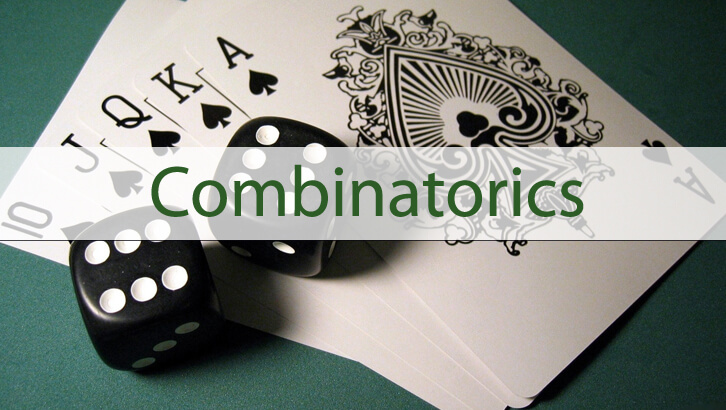# CAT Preparation Online – Permutation and Combination

Question

Product of the distinct digits of a natural number is 60. How many such numbers are possible?

Explanation
60 = 2^2 * 3 * 5
60 cannot be written as a product of two single digit numbers. SO, the number in question should either have 3 or more digits.
Three-digit numbers
The digits could be 345 or 265
Digits being 345 – there are 3! such numbers
There are six numbers for each of these outlines. So, there are 3! + 3! = 12 three-digit numbers
Four-digit numbers
The digits could be 1345, 1265 or 2235.
Digits being 1345 – there are 4! such numbers
Digits being 1265 – there are 4! such numbers
Digits being 2235 – this is not possible as digits have to be distinct.
So, there are totally 24 + 24 four-digit numbers possible. 48 four-digit numbers.

Total number of numbers = 12 + 48 = 60.

(this post has been modified to plug error)